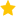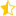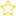Resources for teaching statistics: Classroom Activities, Key Resources

Students often have difficulty identifying experimental units that are non-physical entities.

Experimental units are commonly physical entities such as people, plots in a field, etc.

Tool Makers (an NZAMT assessment resource for Achievement Standard 91583 —  http://www.nzamt.org.nz/ ) and Orbital Express (Teaching Mathematics with Fathom Pg 172) are two examples in which the experimental unit is a non-physical entity.

In Tool Makers we want to compare the distance a ball travels when thrown by a ball-thrower (the tool) for three different length ball-throwers (short, standard and long).  The experimental design is with one student having fifteen throws for each of the three different length ball-throwers.   In order to understand non-physical experimental units we will simplify and compare the shortened length with the standard length.

The difficulty with this design is in identifying the experimental components (treatment, response and in particular the experimental units):

• The treatments here are the two different length ball-throwers, short and standard length.
• The response is the distance thrown in metres.
• What are the experimental units which are being randomly allocated to each treatment group?

In this situation the experimental units are the 30 throws (non-physical entities) and each one of these experimental units (each throw, numbered 1 to 30) is randomly assigned to one of the two treatments, short or standard length.  (This is akin to having 30 people (experimental units) randomly assigned to one of two drugs (treatments).)

This design produces two groups of data – short group and standard group – and these are two independent groups (this is a key point).  A randomisation test can then be conducted on these two independent groups.

You should not pool the results from a number a students in the class.  Each student will have to do this independently.  Pooling of students will not produce independent treatment groups.

In the Orbital Express experiment the treatments are the two types of paper, the response is the distance the paper lands from the marker (cm) and the experimental units are the 14 drops.

Perhaps avoid using experiments which involve non-physical entities especially in the first year of implementing this new standard.

## Rate this resource:(3 ratings)